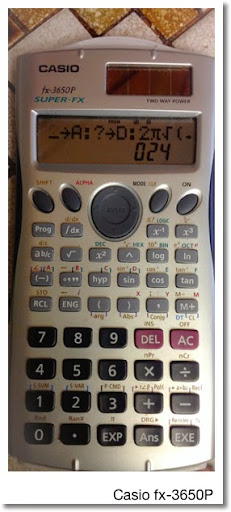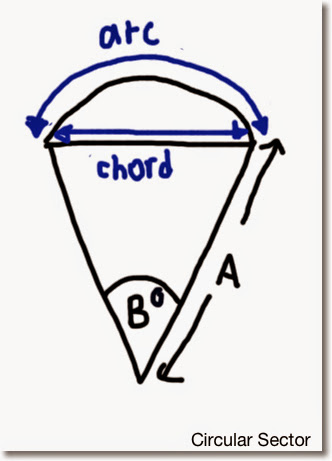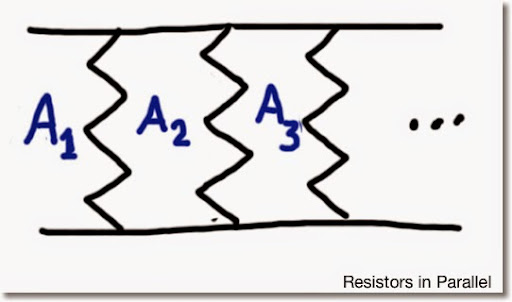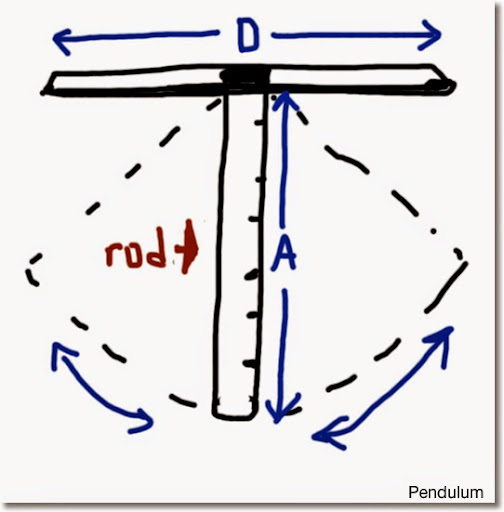## Sunday, May 11, 2014

### Casio fx-3650p: Programming

HAPPY MOTHER'S DAY! Love you Mom! Thanks for all you do - Eddie

Casio fx- 3650p Programs

To celebrate the recent procurement of a Casio fx-5800p, let's dedicate the next several posts to Casio programming calculators. This one is for the fx-3650p, a programming calculator sold world wide (not so much the United States :( ).

* The fx-3650P has only 7 variables (A, B, C, D, X, Y, and M). To save space, variables are recycled.

* You can apply storage arithmetic to memory M, mainly M+ and M-. On the fx-3650P, M+ and M- can be programmable steps. I am not sure if this is possible on programming models (fx-50f, fx-4500p, etc), but this option is not present in Casio's fx-5800p and current graphing calculators (fx-9860, Prizm, etc).

* With Goto and Label, loops can be constructed. The fx-3650p has four comparative tests (=, ≠, >, and ≥).

* Take advantage of not having to close the final parenthesis and begin able to use implicit multiplication to save space.

With that, here are some programs.

Contents:
1. Circular Sectors
2. Stopping Sight Distance
3. Resistors in Parallel
4. Net Present Value
5. Rod Pendulum
6. Vectors: Dot and Cross ProductsCircular Sector

Input:
B = angle in degrees

Formulas:
arc length = 2 * r * sin(θ*π/360)
chord length = (r * θ * π)/180

Program: (28 steps)
? → A : ? → B : Deg :
2 A sin ( B π ÷ 360 ◢ A B π ÷ 180

Example:
A = 3.6
B = 44°

Input: Prog # 3.6 EXE 44 EXE

#: 1, 2, 3, or 4, depending on where your program is stored.

Results (to four decimal points);
0.04825 (chord length) EXE
2.7646 (arc length)

Stoping Sight Distance (U.S. Units)

Input:
A = design vehicle speed in miles/hour
B = grade, as a percentage

Standard constants (acceleration of the car of 11.2 ft/s^2 and 2.5 seconds of reaction time) are used.

Source: Goswami, Indramil Ph.D. P.E. "All In One Civil Engineering PE Breadth and Depth Exam Guide" 2nd Edition. McGraw Hill: 2012

Formula:
SSD = 11/3 * v + (1.075*v^2)/(11.2 + .32G)

Program: (32 steps)
? → A : ? → B :
11 A ⌟ 3 + 1.075 A ² ⌟ ( 11.2 + .32 B

Example 1: 50 mph, grade of 0%
Input: Prog # 50 EXE 0 EXE
Result: 423.4 mph

Example 2: 65 mph, grade of -2%
Input: Prog # 65 EXE -2 EXE
Result: 668.8 mph

The next two programs make use of loops.Total Resistance: Resistors in Parallel

Formula: (1/R1 + 1/R2 + 1/R3 + ... )⁻¹

Note: The fx-3650P allows us to take the M+ to our advantage. Enter each resistor in Ohms. When completed, enter 0 to exit the loop.

Program: (30 steps)
0 → M :
Lbl 0 : ? → A : A = 0 ⇒ Goto 1 :
A ⁻¹ M+ : Goto 0 :
Lbl 1 : M ⁻¹

Example: A circuit has three resistors in parallel of 4 Ω, 3. Ω, and 6 Ω.
Input: Prog # 4 EXE 3 EXE 6 EXE 0 EXE
Result: 1.33333333

Net Present Value

Formulas: Σ (A_M / (1 + B%)^M) for M = 0 to C

Variables:
A = cash flows
B = periodic interest rate
C = number of cash flow (entered in advance)

Calculated Variables:
M = counter
D = total

Program: (51 steps)
? → C : ? → B : 0 → M : 0 → D :
Lbl 0 : ? → A :
D + A ÷ ( 1 + .01 B ) ^ M → D : 1 M+ :
C ≥ M ⇒ Goto 0 : D

Example:
Cash Flow:
CF0 = -\$1,000.00
CF1 = \$0.00
CF2 = \$500.00
CF3 = \$750.00
CF4 = \$1,000.00
Periodic Interest Rate = 10%
Number of Flows = 4 (don't count the initial flow)

Input:
Prog #
4 EXE (number of flows)
10 EXE (periodic Interest rate)
-1000 EXE (cash flows)
0 EXE
500 EXE
750 EXE
1000 EXE

Results: \$659.72 (NPV)Pendulum of the Rod (U.S. units)

(See the above diagram).
A = length of rod
D = length of the board holding the rod

Formulas:
T = 2 π √( I / (m*g*A))
I = inertia of the pendulum
m = mass of the object
g = gravity constant (9.80665 m/s^2, 32.174 ft/s^2)

Using the rod, where I = 1/3 * m * r^2,
T = 2 * π * √(A/96.522) (US units) or
T = 2 * π * √(A/29.41995) (SI units)

Average velocity: V = D/T

Source: Michael Browne, Ph.D. "Schaum's Outlines: Physics for Engineering and Science" 2nd Edition. McGraw Hill, 2010

Program: (24 bytes for US Units)
? → A : ? → D :
2 π √ ( A ÷ 96.522 ◢ D ÷ Ans

Example:
A = 6 ft., D = 1.4 ft
Input: Prog # 6 EXE 1.4 EXE
Results:
1.566543062 sec (T) EXE
0.89368753 ft/sec (V)

Vectors: Dot and Cross Products

Formulas:
Dot Product:
[A, B, C] • [D, X, Y] = A*D + B*X + C*Y
[A, B, C] × [D, X, Y] = [ B*Y-C*X, C*D-A*Y, A*X-B*D ]

Program: (50 bytes)
? → A : ? → B : ? → C :
? → D : ? → X : ? → Y :
A D + B X + C Y ◢
B Y - C X ◢
C D - A Y ◢
A X - B D

Example:
[ 3, 4, 6 ] • [ -1, 2, 3 ] = 23
[ 3, 4, 6 ] × [ -1, 2, 3 ] = [ 0, -15, 10 ]

Input:
Prog # EXE 3 EXE 4 EXE 6 EXE -1 EXE 2 EXE 3 EXE

Results:
23 (dot product) EXE
0 (cross product, x) EXE
-15 (cross product, y) EXE
10 (cross product, z)

Hope that you enjoy these programs, maybe got a few pointers. Have a great day. I will be working with the Casio fx-5800p for my next blog, which I target to put out next week.

Peace!

Eddie

This blog is property of Edward Shore. 2014